### Home > CALC > Chapter 7 > Lesson 7.3.2 > Problem7-123

7-123.
1. Integrate. Homework Help ✎

1.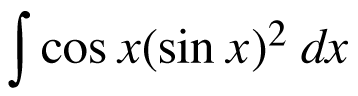2.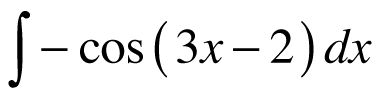3.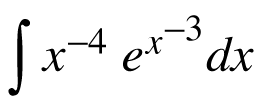4.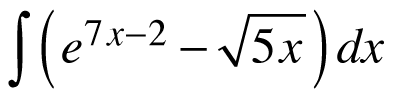5.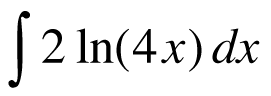6.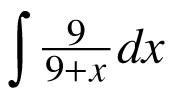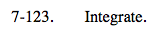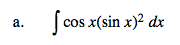Let u = sin(x).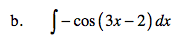ksin(3x − 2) + C
What is k?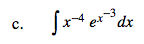Use u-substitution. Careful choosing a u.
Who has the higher power: x−3 or x−4?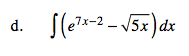Before integrating, rewrite the square root term in the integrand with a fractional exponent.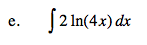$\text{Recall that: }\int \ln(x)dx=x\ln(x)-x+C$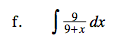$9\int \frac{1}{9+x}dx$